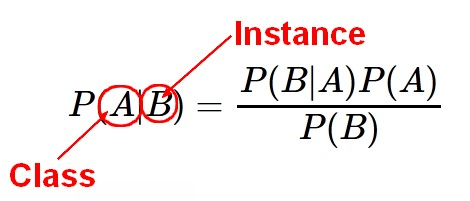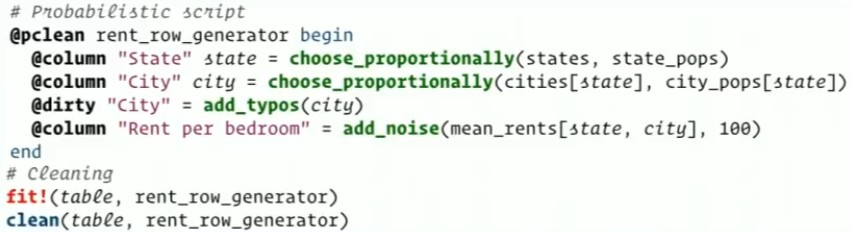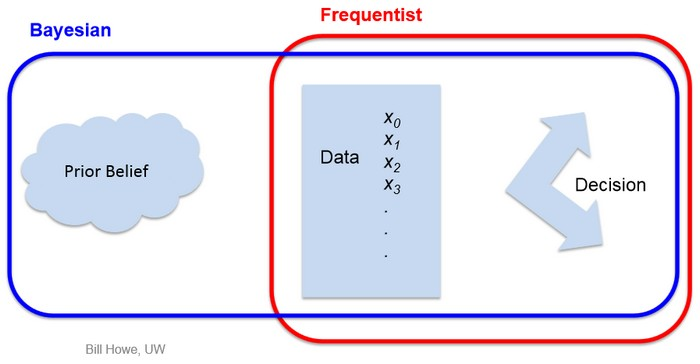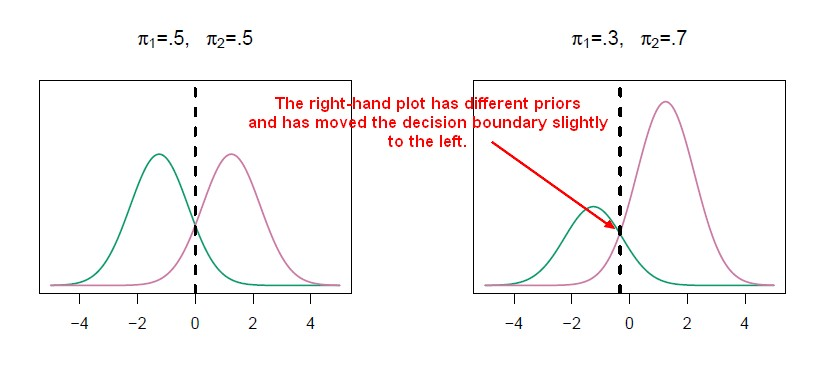# Statistics - Bayes’ Theorem (Probability)

Bayesian probability is one of the different interpretations of the concept of probability and belongs to the category of evidential probabilities.

In the Bayesian view, a probability is assigned to a hypothesis, whereas under the frequentist view, a hypothesis is typically tested without being assigned a probability.

The Bayes theorem defines the probability of the event B and the event A (the evidence or the priori) happening ($P(A \cap B)$ ) with the following formula:

$$P(A \text{ and } B) = P(A) . P(B \text{ after } A) = P(B) . P(A \text{ after } B) \\ P(A \cap B) = P(A|B) . P(B) = P(B|A) . P(A)$$

where:

• $P(A \cap B) = P(A \text{ and } B)$ is the probability of A and B happening.
• $P(A | B) = P(A \text{ after } B)$ is the probability of event A happening given that event B has already happened
• $P(B | A) = P(B \text{ after } A)$ is the probability of event B happening given that event A has already happened
• $P(A)$ is the probability of A happening across the board
• $P(B)$ is the probability of B happening across the board

## Prior

To evaluate the probability of a hypothesis $P(A \cap B)$ , the Bayesian probabilist specifies some prior probability ($P(A)$ of $P(B)$ ).

This prior probability is also known as marginal depending on the probability direction (ie $P(A|B)$ of $P(B|A)$ )

One of its benefit is also its weakness: You have the ability to incorporate prior knowledge but you also need to incorporate prior knowledge.

Different people could use Bayes's Theorem and get different results.

## Formula

From the previous formula, is it possible to deduce the famous formula of Bayes: the Probability of event A happening given that event B has already happened.

$P(A|B) = \fracP(B|A) P(A)}P(B)}$

## Views

Broadly speaking, there are two views on Bayesian probability that interpret the probability concept in different ways. The objective and subjective variants of Bayesian probability differ mainly in their interpretation and construction of the prior probability.

### Objectivist

According to the objectivist view, the rules of Bayesian statistics can be justified by requirements of rationality and consistency and interpreted as an extension of logic.

For objectivists, probability objectively measures the plausibility of propositions, i.e. the probability of a proposition corresponds to a reasonable belief everyone (even a “robot”) sharing the same knowledge should share in accordance with the rules of Bayesian statistics, which can be justified by rationality and consistency.

Many modern machine learning methods are based on objectivist Bayesian principles.

In the objectivist stream, the statistical analysis depends on only the model assumed and the data analysed. No subjective decisions need to be involved. In contrast, “subjectivist” statisticians deny the possibility of fully objective analysis for the general case.

### Subjectivist

According to the subjectivist view, probability quantifies a “personal belief”. For subjectivists, probability corresponds to a 'personal belief'. For subjectivists, rationality and coherence constrain the probabilities a subject may have, but allow for substantial variation within those constraints.

## Example

### Example 1: Getting cards out of a deck

Probability of getting 2 kings out of a deck of cards.

$$Pr(\text{2 kings}) = \frac{4}{52} * \frac{3}{51} \approx 0.45 \%$$

• Prior Probability: There is 4 kings in the set of 52 cards
• Second Probability: There is only 3 kings in a set of 51 cards

### Example 2: Breast cancer Probability

#### Problem

• 1% of women at age forty who participate in routine screening have breast cancer.
• 80% of women with breast cancer will get positive mammographies.
• 9.6% of women without breast cancer will also get positive mammographies.

A woman in this age group had a positive mammography in a routine screening. What is the probability that she actually has breast cancer?

#### Groups Form

To put it another way, before the mammography screening, the 10,000 women can be divided into two groups:

Group 1:    100 women with breast cancer.
Group 2:  9,900 women without breast cancer.
Total:   10,000 patients



After the mammography, the women can be divided into four groups:

Group A:     80 women with breast cancer, and a positive mammography.
Group B:     20 women with breast cancer, and a negative mammography.
Group C:    950 women without  breast cancer, and a positive mammography.
Group D:  8,950 women without breast cancer, and a negative mammography.
Total  : 10,000 patients



#### Two grid event Forms

Two grid form with event A, event B, and not A and not B.

 Event A Non Event A (~A) Have Cancer (1%) Do not have cancer (99%) True Positive: 1% x 80% False Positive: 99% x 9.6% False Negative: 1% x 20% True Negative: 99% x 90.4%

#### Solution

$$\begin{array}{rrl} P(cancer) & = & 0.01 \\ P(\text{positive test | cancer}) & = & 0.8 \\ P(\text{positive test}) & = & P(\text{positive test} | cancer) * P(cancer) + P(\text{positive test} | no cancer) * P(\text{no cancer}) \\ P(\text{positive test}) & = & 0.8 * 0.01 + 0.096 * 0.99 = 0.103 \\ P(cancer | \text{positive test}) & = & \fracP(\text{positive test} | cancer) P(cancer)}P(\text{positive test})} \\ P(cancer | \text{positive test}) & = & \frac0.8 * 0.01}0.103} = 0.078 \end{array}$$

## Documentation / Reference

Discover More(Machine learning|Inverse problems) - Regularization

Regularization refers to a process of introducing additional information in order to: solve an ill-posed problem or to prevent overfitting. This information is usually of the form of a penalty...Data - Uncertainty

How likely is this prediction to be true? probability distributionconfidence interval3055303Erik Meijer - Making Money Using Math Thomas Bayes anticipated the need for dealing with uncertainty and formulated...Data Mining - Naive Bayes (NB)

Naive Bayes (NB) is a simple supervised function and is special form of discriminant analysis. It's a generative model and therefore returns probabilities. It's the opposite classification strategy...Data Quality - Data (cleaning|scrubbing|wrangling)

Data cleansing or data scrubbing is the act of: detecting (ie data validation) and correcting (or removing) corrupt or inaccurate records from a record set, table, or database. Used mainly in...Machine Learning - (Baseline|Naive) classification (Zero R)

A baseline classification uses a naive classification rule such as : Base Rate (Accuracy of trivially predicting the most-frequent class). (The ZeroR Classifier in Weka) always classify to the largest...Statistics - Probability (of an event) / Likelihood

The probability of an event is the subjective chance that it will happen. The laws of probability are heavily build with therandom notion. A probability is a non-negative number between 0 and 100%. ...Statistics Learning - Discriminant analysis

Discriminant analysis is a classification method. In discriminant analysis, the idea is to: model the distribution of X in each of the classes separately. use what's known as Bayes theorem to flip...